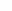COSC 1415 Practice Test #2
conditions, while loops, for loops

Using the variable declarations below, what will the following conditions evaluate to?

int Apple = 5, Pear = 8, Grape = -4;

1.(Apple > Grape)

2.(Pear > Grape && Apple < Pear)

3.(Grape != 3 || Pear != 8)

4.(!(Apple >= Apple) && !(Grape == (Pear - 12)))

5.(Pear = Grape)

How many iterations will the following for loops execute?

6.for (int X=1; X<=3; X++)

7.for (int X=5; X>=0; X--)

8.for (int G=0; G<8; G++)

9.for (int Yellow=1; Yellow<=20; Yellow=Yellow+2)

10.for (int X=5; X>8; X++)

11.What C++ statement will allow your program to show the calendar for 2008?

What will the following assignment statements evaluate to assuming the variable declarations below?

int J = 1, K = 0, L = -1, M = 4, N = 3;

 Assignment Statement Resulting Value of the Variable Q Q = 8 % M + 15; 12.select 0 1 5 8 15 20 Q = 2 + 2 * M / 2 - 2; 13.select 0 4 6 Q = N * 2 + M * 4 - J / J; 14.select 0 11 22 33 20 21 Q = (N * 8) % 5 - L; 15.select 0 -1 -4 1 4 5

Here's some more difficult problems to really test your skill:

 float A = 1, B = 10; while (A < 10) {    if (A == B) cout << A;    A++;    B = B - 0.5; }  16.What will this output? select 5 6 7 8

 for (int X=99; X>1; X--) {    if (X==4 || X == 3) cout << X * 2; }  17.What will this code output? select 8 86 68 169

 for (int X=1; X<100; X=X*2) {    if (X == 10) cout << "yes";    if (X == 20) cout << "no"; } 18.What will this code output? select yes no yesno128

 for (int Y=1; Y<5; Y=Y+2) {     cout << Y-1; } 19.What will this code output?  select 02 13 024 1234

 int Apple=5, Pear=0; while (Pear != Apple) {   cout << Pear;   Apple = Apple - (Pear + 1);   Pear = Pear + 1; } 20.What does this code output?  select 02 01 024 0011

 bool G = true; short int F = 0; while (G) {   cout << F + 1;   if (F > 5)     G = false;   F++; } cout << F + 1;21.What will this output? select 12345678 1234567 0123456 01234567

 float J = 1.5; if (J > 2)   cout << "J";   cout << "K";22.What will this output? select J K JK

You got out of correct. Your Score: %